# [EEP 147]: Introduction to Programming and the ESGAES California Power Plant - Huntington Beach, CA

# Python Basics

In this notebook, we will go over simple techniques in Python and Matplotlib that you can use to generate graphs that will help you in analyzing the ESG!

First on our agenda is to import dependencies -- or packages in Python that add to the basic functions in Python. Kind of like accessorizing! For example, matplotlib allows us to generate the graphs we will be using.

The format is as follows: from (package) import (stuff), where the "stuff" we're importing can range from a specific function in that package to a whole library of functions, as is the case when we type import (package) as (name).

In :
from datascience import *
import matplotlib.pyplot as plt
%matplotlib inline
import numpy as np
import pandas as pd
plt.style.use('fivethirtyeight')


## Section 1: Math in Python

Python is the programming language that we will use in this lab. Although this lab will go over some basics, should you be more interested in learning Python feel free to check out the following resources:

Mathematical Expressions In Python, we can carry out all the mathematical processes you know and love:

• Add using +
• Subtract using -
• Multiply using *
• Divide using /
• Exponentiate using **
• Floor divide using //
• Take the remainder / modulo using %

The most of these you should be familiar with, but let's go over some of the more obscure processes while beginning to implement some python code!

To run the code in the following cells, press Shift + Enter/Return!

In :
# So what exactly does floor divide do?
10 // 3

Out:
3
In :
# What about modulo?
10 % 3

Out:
1

Very cool! Now we'll let you try, and notice that we can use parentheses to organize our order of operations.

Exercise: Take the product of three and three to the power of six and subtract 168.

In :
# Insert your code where the dots are:
...


Awesome job! Feel free to add more cells using the + button in the upper left hand corner of the lab and play around with more mathematical expressions later! In the meantime, let's move on to the next section.

## Section 2: Variables

As you might recall, a name that is used to denote a value is called a variable. In python, variables can be declared and values can be assigned. Here are a few examples of variables and their assignment:

In :
x = 2
m = 3
b = 4
y = m*x + b
# Look familiar? Press shift + enter to see the value!
y

Out:
10

Output and Printing

As you might have noticed at the end, there is a difference between returning and printing:

• Return: A value that is not necessarily printed, but is stored away inside a computer if we assign or bind it to a name.
• Printing: A value that pops up on our screen. We print using a print function and return a value using the return function.

Functions?

You might recall that a function receives input and correspondingly will output something. In Python, we have numerous functions, such as:

• print: The command print('hi') will print 'hi' out to the screen.
• sum: The command sum(2,3,4) will sum up the values enclosed in the parentheses and return the value.
• And more!

The best thing about functions is that, in Python, we can make our own functions! We will discuss this more in depth later, but for now just remember that to call a function, we write the name of the function, like print() and we place our arguments inside the parentheses.

Let's try it for ourselves!

Exercise: Try printing out the phrase 'Hello World!'

In :
...

Out:
Ellipsis

## Section 3: Functions and Loops

A function is a block of organized, reusable code that is used to perform a single, related action. Take for example a factorial, denoted x!, which takes the initial value x and multiplies it by x-1 and x-2 and so on and so forth until it gets to 1! Typing this all out would look something like this:

In :
# Let's pick a random value for x:
x = 5
factorial = 5 * 4 * 3 * 2 * 1
factorial

Out:
120

This might not seem too troublesome now, but imagine doing this by hand for a larger number like 123! Instead, let's consider writing a function that can take in any value (such as 123) and output the factorial!

Function Structure

So how can we begin writing a function? Well there is a very simple structure to them:

**def** function_name(arguments): [function procedures] **return** [output]

There are some aspects of a function that are required no matter what kind of function you are writing. You will always begin writing a function by writing def, followed by the name of your function. Following the name of your function, you will want to specify your inputs by using parentheses and giving your inputs names. These names can be anything you'd like, but generally you'd like them to be memorable and symbolic of what you're trying to do.

Before typing in your functions procedure in the body of your function, you'll want to end the first line with a :. Then you're ready to proceed to the body and second line of your function! You will want to indent (press tab or space 4 times) and write what you'd like your function to do.

Lastly, you'll want to end the function by writing what you'd like your function to return.

Example: Let's look at what a factorial function would look like!

In :
def factorial_func(x):
product = 1
while x > 0:
product = product * x
x = x - 1
return product

# Now let's test out our new factorial function!
factorial_func(5)

Out:
120
In :
# Try calculating the factorial value for the big number from before:
factorial_func(1)

Out:
1

Amazing! However, you might have noticed there were some new features used, which brings us to our next small topic.

Loops

Something that came in handy for this equation was a loop. A loop is a piece of code that repeats a block of code while a condition is true or for a certain number of times. Like we just not-so-subtly hinted, there are two very important kinds of loops: for loops and while loops. In the case of our function above, the code under the while loop was repeated while x > 0. On the other hand, a for loop will continue looping for a specified number of times.

## Section 4: Data Structures

So now that we know how to calculate things and create functions to do so, how can we organize large amounts of information?

The solution to our problem is a data structure! A data structures is simply a means by which to contain and organize our data or information. They include:

• List: A list holds an ordered collection of items similar to a grocery list.
• Dictionary: Like an addressbook in which keys are associated with values (similar to names and phone numbers in addressbooks).
• Set: An unordered collection of items, and they operate similar to how Venn Diagrams do.

Here is how we can use lists:

In :
# Creating a list using brackets and commas in between:
names = ['Helen', 'Nadeem', 'Alma', 'Nika']
names

Out:
['Helen', 'Nadeem', 'Alma', 'Nika']
In :
# The first name in our list, located at position 0:
names

Out:
'Helen'
In :
# Adding a name, feel free to change the name to yours!
names.append('Sam')
names

Out:
['Helen', 'Nadeem', 'Alma', 'Nika', 'Sam']

Exercise: Now you try creating a list with the names of some of your friends or pets!

In :
# Create your list below:
...


As opposed to a list (or array), a dictionary contains keys and values that, when defined, are separated by a colon. Take a look at the example below:

In :
# Creating a dictionary
dictionary = {'Helen': 'Math', 'Nadeem': 'Physics', 'Alma': 'Data Science', 'Nika': 'MCB'}


To call a certain value in a dictionary, we use the corresponding key:

In :
dictionary['Helen']

Out:
'Math'

Calling an index won't work here! Feel free to play around with the dictionary or create your own. One thing to note is that in a given dictionary, the keys must be unique, but values do not have to be.

In addition to the data structures listed above, we can also organize our information in a table. Similar to Google Sheets or Microsoft Excel, we will be organzing our data into nice-looking tables.

## Section 5: Tables

In this section, we'll cover some basic table functions. In order to begin filtering through information stored in a table, we'll have to "read in" the information. Most of the time, information to be displayed as a table is stored as a .csv file which stands for comma separated values.

To read in a file, we use the following command:

Table.read_table('file_name.csv')

and in order to store it, we'll assign it a name or label. We'll begin by reading in the file that you'll be using for the remainder of the lab:

In :
# Just run this code block!

In :
ESG_table.show(5)

Group Group_num UNIT NAME Capacity_MW Heat_Rate_MMBTUperMWh Fuel_Price_USDperMMBTU Fuel_Cost_USDperMWH Var_OandM_USDperMWH Total_Var_Cost_USDperMWH Carbon_tonsperMWH FixedCst_OandM_perDay Plant_ID
Big Coal 1 FOUR CORNERS 1900 11.67 3 35 1.5 36.5 1.1 $8,000 11 Big Coal 1 ALAMITOS 7 250 16.05 4.5 72.22 1.5 73.72 0.85$0 12
Big Coal 1 HUNTINGTON BEACH 1&2 300 8.67 4.5 39 1.5 40.5 0.46 $2,000 13 Big Coal 1 HUNTINGTON BEACH 5 150 14.44 4.5 65 1.5 66.5 0.77$2,000 14
Big Coal 1 REDONDO 5&6 350 8.99 4.5 40.44 1.5 41.94 0.48 $3,000 15 ... (37 rows omitted) Table Manipulations One of the many manipulations you can make on a table is to sort it by some value. When do you think this might be helpful? In order to sort, we will use the following table method: table.sort("column_to_sort_by", descending = False) in which table is the table you are working with, .sort is the table method, and "column_to_sort_by" is the column label that you'd like to use when sorting your table. The label must be placed in quotation marks as it is a string or phrase. Lastly, an optional second command can be passed in following the comma. Something important to note is the second entry in the table method table.sort. This second argument is optional and decides whether the table will be sorted in either the ascending or descending manner. You are perfectly able to use the table.sort method without specifying an order. The following code sorts the different groups in ascending order by their Total_Var_Cost and assigns this sorted table to a new name. Here we've specified ascending order manually, however the default for the method, given that you didn't specify anything will always be descending = False. In : ESG_sorted = ESG_table.sort("Total_Var_Cost_USDperMWH", descending = False)  In : # Run this code block to view the sorted table; compare it to the first one that we looked at: ESG_sorted.show(5)  Group Group_num UNIT NAME Capacity_MW Heat_Rate_MMBTUperMWh Fuel_Price_USDperMMBTU Fuel_Cost_USDperMWH Var_OandM_USDperMWH Total_Var_Cost_USDperMWH Carbon_tonsperMWH FixedCst_OandM_perDay Plant_ID Old Timers 7 BIG CREEK 1000 nan 0 0 0 0 0$15,000 61
Fossil Light 8 HELMS 800 nan 0 0 0.5 0.5 0 $15,000 72 Fossil Light 8 DIABLO CANYON 1 1000 1 7.5 7.5 4 11.5 0$20,000 75
Bay Views 4 MOSS LANDING 6 750 6.9 4.5 31.06 1.5 32.56 0.37 $8,000 33 Bay Views 4 MOSS LANDING 7 750 6.9 4.5 31.06 1.5 32.56 0.37$8,000 34

... (37 rows omitted)

So we've seen how to sort in ascending order, but what if we wanted the most expensive group first? We can simply run the same command but use the optional input, descending = True. Try it out in the code block below:

In :
# Replace the ellipsis below with the correct command:
ESG_table.sort("Total_Var_Cost_USDperMWH", ... )

Out:
Group Group_num UNIT NAME Capacity_MW Heat_Rate_MMBTUperMWh Fuel_Price_USDperMMBTU Fuel_Cost_USDperMWH Var_OandM_USDperMWH Total_Var_Cost_USDperMWH Carbon_tonsperMWH FixedCst_OandM_perDay Plant_ID
Big Gas 2 KEARNY 200 19.9 4.5 89.56 0.5 90.06 1.06 $0 26 Fossil Light 8 HUNTERS POINT 4 250 16.53 4.5 74.39 1.5 75.89 0.88$1,000 74
Beachfront 5 ELLWOOD 300 16.69 4.5 75.11 0.5 75.61 0.89 $0 44 Big Coal 1 ALAMITOS 7 250 16.05 4.5 72.22 1.5 73.72 0.85$0 12
East Bay 6 POTRERO HILL 150 15.41 4.5 69.33 0.5 69.83 0.82 $0 56 Big Coal 1 HUNTINGTON BEACH 5 150 14.44 4.5 65 1.5 66.5 0.77$2,000 14
Big Gas 2 NORTH ISLAND 150 14.44 4.5 65 0.5 65.5 0.77 $0 24 Beachfront 5 ETIWANDA 5 150 13.64 4.5 61.39 1.5 62.89 0.72$1,000 43
Bay Views 4 OAKLAND 150 13.48 4.5 60.67 0.5 61.17 0.72 $0 35 East Bay 6 PITTSBURGH 7 700 13.16 4.5 59.22 0.5 59.72 0.7$4,000 53

... (32 rows omitted)

There are a wide variety of table methods, but here are the highlights, followed with examples:

• table.where(column, value_you_want), where column is the column you'd like to select from and value_you_want is the item you're searching for. The output will be a table that only contains elements that are the value you want for the column you specified.
• table.column(column), where column is again the column you'd like to select. However, this method returns the entire column as an array of the items in that column!

Note that when specifying a column, you can use either the string label or the index of the column. And don't forget that in python, we begin counting (or indexing) at 0. Below are some examples:

In :
Big_Coal= ESG_sorted.where("Group","Big Coal")
Big_Coal

Out:
Group Group_num UNIT NAME Capacity_MW Heat_Rate_MMBTUperMWh Fuel_Price_USDperMMBTU Fuel_Cost_USDperMWH Var_OandM_USDperMWH Total_Var_Cost_USDperMWH Carbon_tonsperMWH FixedCst_OandM_perDay Plant_ID
Big Coal 1 FOUR CORNERS 1900 11.67 3 35 1.5 36.5 1.1 $8,000 11 Big Coal 1 HUNTINGTON BEACH 1&2 300 8.67 4.5 39 1.5 40.5 0.46$2,000 13
Big Coal 1 REDONDO 5&6 350 8.99 4.5 40.44 1.5 41.94 0.48 $3,000 15 Big Coal 1 REDONDO 7&8 950 8.99 4.5 40.44 1.5 41.94 0.48$5,000 16
Big Coal 1 HUNTINGTON BEACH 5 150 14.44 4.5 65 1.5 66.5 0.77 $2,000 14 Big Coal 1 ALAMITOS 7 250 16.05 4.5 72.22 1.5 73.72 0.85$0 12

But perhaps you're interested in a specific group? In the code block below, select what group you'd like to take a closer look at?

In :
# REPLACE '...' with your specific group! Remember that the input should be a string, so don't remove the quotes!
selection = 'Big Coal'
Group = ESG_sorted.where("Group", selection)


From the original table, we extract information about one particular group: Big Coal. In the following code blocks we create 2 arrays, width_coal and height_coal. The items of the width_coal array are basically the capacity of Big Coal plants in MWH while height_coal contains their cost in USD per MWH.

In :
# Here we select the appropriate columns:
width_group = Group.column("Capacity_MW")
height_group = Group.column("Total_Var_Cost_USDperMWH")

# Don't worry about the following code, we are simply making it 'look nice':
print("width_coal: ", width_group)
print("height_coal: ", width_group)

width_coal:  [1900  300  350  950  150  250]
height_coal:  [1900  300  350  950  150  250]


### Congratulations! You've successfully completed the Intro to Python section!

We will now move onto the application of these techniques. Make sure you understand the tables we created as we will be using these in the parts that follow.

# Application to the Electricity Strategy Game

Next, we will use the widths we generated from the sorted ESG table and create an array of x positions used to graph the Variable Cost vs Capacity_MW bar graph with the find_x_pos function.

In :
def find_x_pos(widths):
cumulative_widths = 
cumulative_widths.extend(np.cumsum(widths))
half_widths = [i/2 for i in widths]
x_pos = []
for i in range(0, len(half_widths)):
x_pos.append(half_widths[i] + cumulative_widths[i])
return x_pos

In :
new_x_group = find_x_pos(width_group)
new_x_group

Out:
[950.0, 2050.0, 2375.0, 3025.0, 3575.0, 3775.0]

Now we make a bar plot of the data we have collected so far, with new_x_coal on the x axis and height_coal on the y axis.

In :
# Make the plot
plt.figure(figsize=(9,6))
plt.bar(new_x_group, height_group, width=width_group, edgecolor = "black")
# Add title and axis names
plt.title(selection)
plt.xlabel('Capacity_MW')
plt.ylabel('Variable Cost')

plt.show()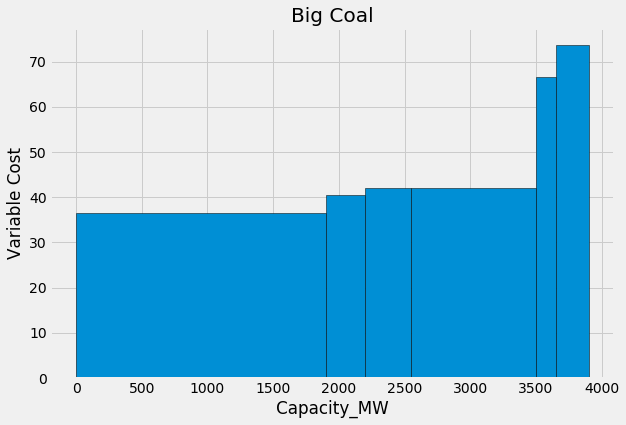Repeat the same process, this time for all the energy sources. Since we are not concerned with any one particular group here, we use the original ESG_sorted table.

In :
width = ESG_sorted.column("Capacity_MW")
width
height = ESG_sorted.column("Total_Var_Cost_USDperMWH")
height

Out:
array([ 0.  ,  0.5 , 11.5 , 32.56, 32.56, 34.5 , 34.5 , 36.5 , 36.61,
36.61, 38.06, 38.06, 38.78, 39.06, 39.5 , 40.5 , 40.94, 41.22,
41.67, 41.94, 41.94, 42.39, 42.67, 43.83, 44.83, 47.44, 49.17,
49.61, 52.06, 52.5 , 53.94, 58.28, 59.72, 61.17, 62.89, 65.5 ,
66.5 , 69.83, 73.72, 75.61, 75.89, 90.06])
In :
new_x = find_x_pos(width)

In :
# Make the plot
plt.figure(figsize=(9,6))
plt.bar(new_x, height, width=width, edgecolor = "black")
#plt.xticks(y_pos, bars)
# Add title and axis names
plt.title('All Energy Sources')
plt.xlabel('Capacity_MW')
plt.ylabel('Variable Cost')

plt.show()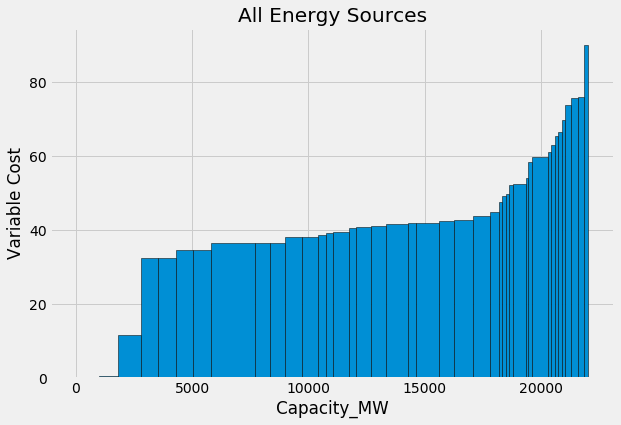Our aim now is to make a plot which shows all the different groups with unique colors. The first step in doing this is creating a dictionary called energy_colors_dict in which the groups and colors are a key-value pair. We use the following code to accomplish this:

In :
energy_colors_dict = {}
count = 0
colors = ['#EC5F67', '#F29056', '#F9C863', '#99C794', '#5FB3B3', '#6699CC', '#C594C5']
for i in set(ESG_sorted['Group']):
energy_colors_dict[i] = colors[count]
count += 1


Now, we just map the colors from our dictionary to a series which contains all the groups. Our resultant list will have the same length as the ESG_sorted table.

In :
colors_mapped = list(pd.Series(ESG_sorted['Group']).map(energy_colors_dict))
ESG_sorted = ESG_sorted.with_column('Color', colors_mapped)


Our plot now shows the Variable Cost and Capacity for each group in a different color.

In :
# Make the plot
plt.figure(figsize=(9,6))
plt.bar(new_x, height, width=width, color=ESG_sorted['Color'], edgecolor = "black")
#plt.xticks(y_pos, bars)
# Add title and axis names
plt.title('All Energy Sources')
plt.xlabel('Capacity_MW')
plt.ylabel('Variable Cost')
plt.legend()
plt.show()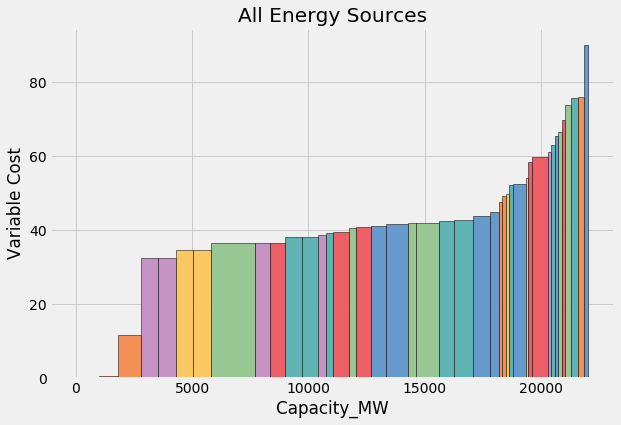To make sense of which color corresponds to which group, we make a plot that can serve as a legend for our colors.

In :
plt.figure(figsize=(5,1))
plt.bar(energy_colors_dict.keys(), 1, color = energy_colors_dict.values())
plt.xticks(rotation=60)
plt.title('Legend')
plt.show()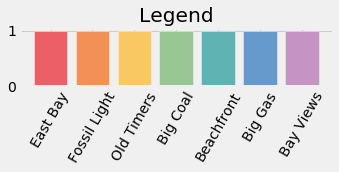## Prediction¶

In order to determine the market price of energy in our simulation, we rely on the graphs we produced above, but we're missing one key factor: demand. We don't know exactly how much energy will be demanded in a given frame of time, however we can make predictions based off of estimates that we are given, and use those predictions to calculate the profitability of our plants.

We can set an estimated demand below.

In :
demand = 20000


The functions below will calculate the maximum variable cost companies can have in order to make profit based on the demand above. For now, we will make the assumption that plants are willing to sell at a price equal to their variable cost.

In :
def price_calc(demand, sorted_table):
price = 0
sum_cap = 0
for i in range(0,len(sorted_table['Capacity_MW'])):
if sum_cap + sorted_table['Capacity_MW'][i] > demand:
price = sorted_table['Total_Var_Cost_USDperMWH'][i]
break
else:
sum_cap += sorted_table['Capacity_MW'][i]
price = sorted_table['Total_Var_Cost_USDperMWH'][i]
return price

In :
price = price_calc(demand, ESG_sorted)
price

Out:
59.72
In :
def price_line_plot(price):
plt.axhline(y=price, color='r', linewidth = 2)
print("Price: " + str(price))

In :
def demand_plot(demand):
plt.axvline(x=demand, color='r', linewidth = 2)
print("Capacity: " + str(demand))


Next we will add the vertical line for demand and horizontal line for variable cost cap into the graph. Since we have our plants graphed in order of lowest variable cost to highest variable cost, we can see that the companies to the left of the vertical demand line will produce energy while the companies to the right of the vertical demand line will not. This is because the public will purchase from the plants that have the cheapest prices, and we have graphed the cumulative energy production of companies ordered by increasing variable cost of production.

In :
# Make the plot
plt.figure(figsize=(9,6))
plt.bar(new_x, height, width=width, color=ESG_sorted['Color'], edgecolor = "black")
plt.title('All Energy Sources')
plt.xlabel('Capacity_MW')
plt.ylabel('Variable Cost')
price_line_plot(price)
demand_plot(demand)

plt.show()

Price: 59.72
Capacity: 20000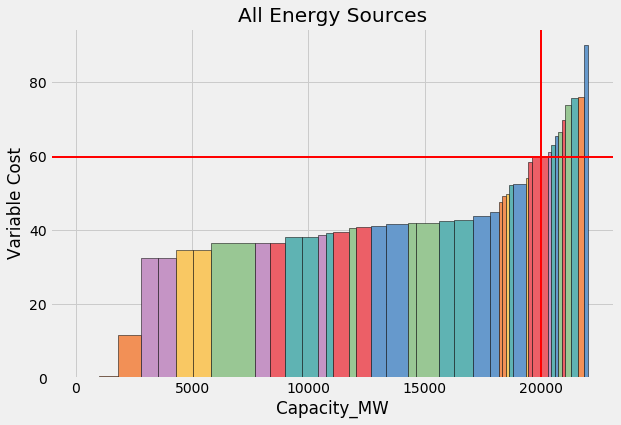Now we will graph our variable cost cap with just the Big Coal plants.

In :
# Make the plot
plt.figure(figsize=(9,6))
plt.bar(new_x_group, height_group, width=width_group, edgecolor = "black")
plt.title(selection)
plt.xlabel('Capacity_MW')
plt.ylabel('Variable Cost')
price_line_plot(price)

plt.show()

Price: 59.72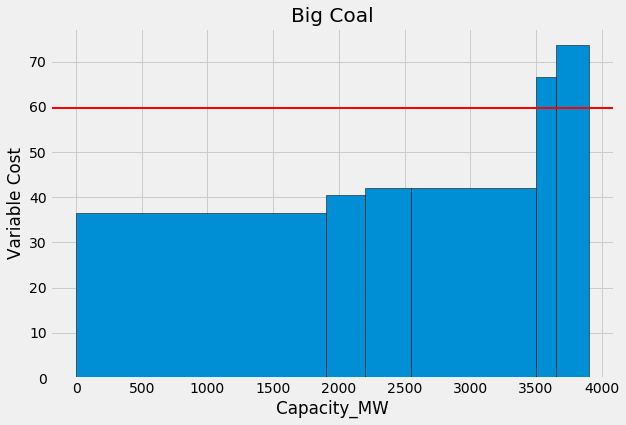Lastly, we calculate the profit that our plants can make. Here we first calculate the revenue for the plant, by multiplying the capacity that will be produced by the market price. Next, we subtract the cost of production for each plant that is operating, and get our estimate for profit!

In :
sum(Group.where("Total_Var_Cost_USDperMWH", are.below(price))["Capacity_MW"])

Out:
3500
In :
def profit(sorted_table, price):
capacity_subset = sum(sorted_table.where("Total_Var_Cost_USDperMWH", are.below(price))["Capacity_MW"])
revenue = capacity_subset * price
cost = 0
for i in range(len(sorted_table.where("Total_Var_Cost_USDperMWH", are.below(price))["Total_Var_Cost_USDperMWH"])):
cost += sorted_table.where("Total_Var_Cost_USDperMWH", are.below(price))["Total_Var_Cost_USDperMWH"][i]\
* sorted_table.where("Total_Var_Cost_USDperMWH", are.below(price))["Capacity_MW"][i]
return revenue - cost

In :
profit(Group, price)

Out:
72998.0

So now we have the ability to estimate the amount of profit our plants will generate based on a given amount of demand. However, there is a caveat in what we have done. The graphs above are generated by using the marginal cost. In reality, you (and every other team) can choose to price their plants however they wish, so there is no guarantee that any of these estimates are accurate.

# Conclusion and Resources¶

Congratulations! You have completed your Jupyter Notebook tutorial for the ESG. We hope that this resource proves useful to you throughout the course of the game. If you do have questions, please do not hesitate to reach out and ask anyone from the modules team via Piazza or email, as we are here to help.

Module Developers: Alec Kan ([email protected]), Alma Pineda, Aarish Irfan, Elaine Chien, and Octavian Sima.

Data Science Modules: http://data.berkeley.edu/education/modules

In [ ]: